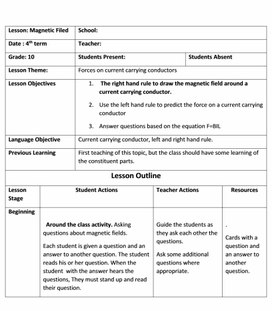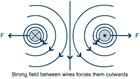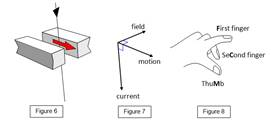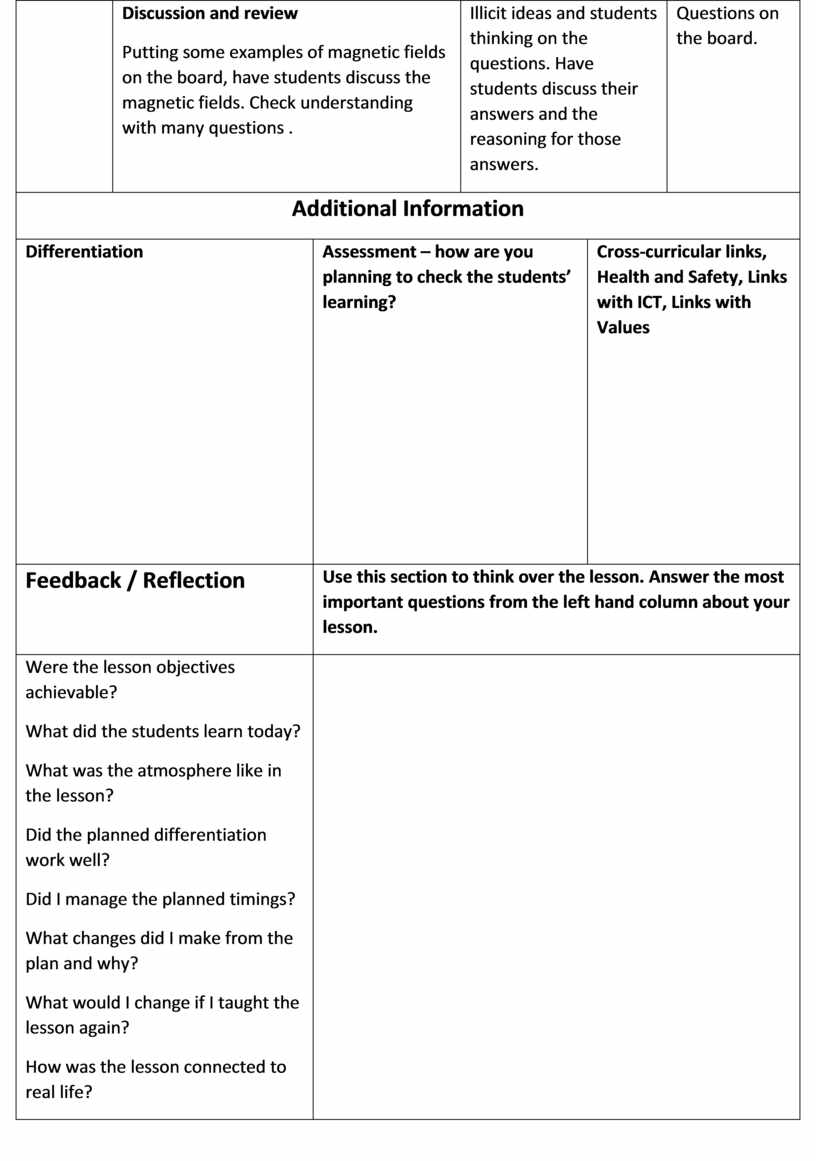Forces on current carrying conductors
Оценка 4.8

# Forces on current carrying conductors

Оценка 4.8
doc
07.05.2020Forces on current carrying conductors.doc

 Lesson: Magnetic Filed School: Date : 4th term Teacher: Grade: 10 Students Present: Students Absent Lesson Theme: Forces on current carrying conductors Lesson Objectives The right hand rule to draw the magnetic field around a current carrying conductor.   Use the left hand rule to predict the force on a current carrying conductor Answer questions based on the equation F=BIL Language Objective Current carrying conductor, left and right hand rule. Previous Learning First teaching of this topic, but the class should have some learning of the constituent parts. Lesson Outline Lesson Stage Student Actions Teacher Actions Resources Beginning Around the class activity. Asking questions about magnetic fields. Each student is given a question and an answer to another question. The student reads his or her question. When the student  with the answer hears the questions, They must stand up and read their question. Guide the students as they ask each other the questions. Ask some additional questions where appropriate. . Cards with a question and an answer to another question. Middle (Main activities) Demonstration and Questions Students watch a demonstration to show the force on two parallel conductors Right hand rule: After some direction. Students should draw the magnetic field around wires coming into and out of a page.Students should use the magnetic field to explain the repulsive and attractive forces a acting on the two wires. Perform demonstration and show students the right hand rule.   Guide students as they answer questions.   Discuss students ideas and get students to predict what will happen if the current is reversed or if the current is lower. Equipment for demonstration.   Questions. Middle (Main activities) Left hand rule and questions on F=BIL Use the magnetic field to predict the force on a current carrying conductor through a  magnetic field. Demonstration: Carry out a demonstration with a current carrying conductor in a magnetic field. Ask students. What happens if we: Change the direction of the current, Increase the size of the current, Change the direction of the magnet. Varify their prediction and discuss their ideas. Questions: Students use the magnetic fieldFollow with questions on the equation. F=BI Discuss the left hand rule and use the magnetic field around a wire to help students predict the direction of the force on a wire through a magnetic field.   Carry out a demonstration and ask students questions. Questions. Board and Demonstration. Equipment for demonstration. Discussion and review Putting some examples of magnetic fields on the board, have students discuss the magnetic fields. Check understanding with many questions . Illicit ideas and students thinking on the questions. Have students discuss their answers and the reasoning for those answers. Questions on the board. Additional Information Differentiation Assessment – how are you planning to check the students’ learning? Cross-curricular links, Health and Safety, Links with ICT, Links with Values Feedback / Reflection Use this section to think over the lesson. Answer the most important questions from the left hand column about your lesson. Were the lesson objectives achievable? What did the students learn today? What was the atmosphere like in the lesson? Did the planned differentiation work well? Did I manage the planned timings? What changes did I make from the plan and why? What would I change if I taught the lesson again? How was the lesson connected to real life?

## Discussion and review Putting some examples of magnetic fields on the board, have students discuss the magnetic fieldsСкачать файл